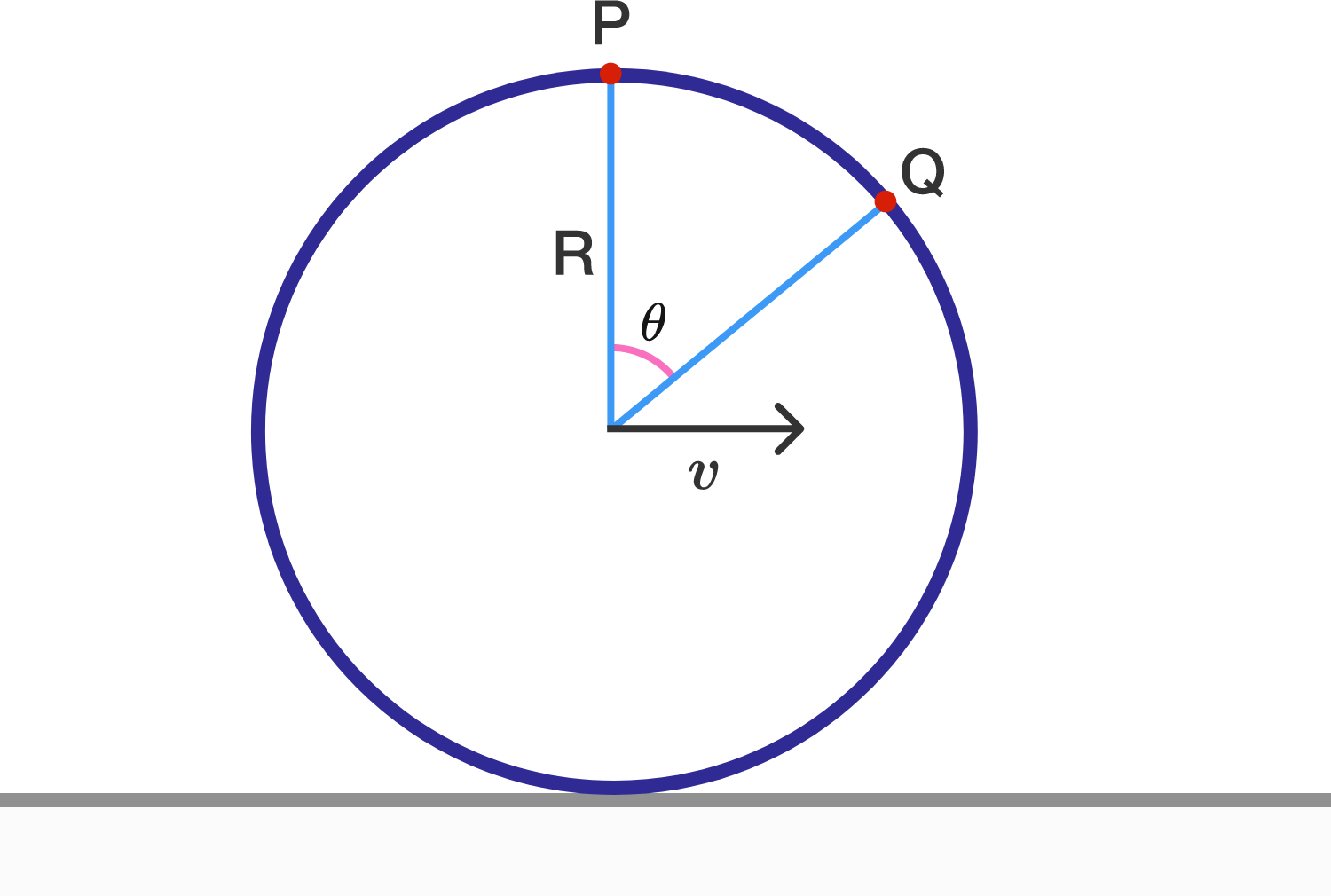# Complexity Of Frame ChangesA ring of radius $R$ is rolling without slipping on a horizontal surface at a linear speed of $v$.

Let $P$ and $Q$ be two points on the ring, as shown in the figure above.

Find the radius of curvature of the path traveled by $P$ as observed from $Q$.

Details and Assumptions:

• Take $R = 10\text{ m}, v = 25 \text{ m/s},$ and $\theta = 30^{\circ}$.
×

Problem Loading...

Note Loading...

Set Loading...